# GMAT Math : DSQ: Calculating the length of the side of a square

## Example Questions

### Example Question #1 : Dsq: Calculating The Length Of The Side Of A Square

Is Rectangle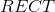a square?

Statement 1: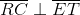Statement 2: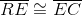Statement 2 ALONE is sufficient to answer the question, but Statement 1 ALONE is NOT sufficient to answer the question.

BOTH statements TOGETHER are insufficient to answer the question.

BOTH statements TOGETHER are sufficient to answer the question, but NEITHER statement ALONE is sufficient to answer the question.

EITHER statement ALONE is sufficient to answer the question.

Statement 1 ALONE is sufficient to answer the question, but Statement 2 ALONE is NOT sufficient to answer the question.

EITHER statement ALONE is sufficient to answer the question.

Explanation:

A rectangle, by definition, is a parallelogram. Statement 1 asserts that the diagonals of this parallelogram are perpendicular. Statement 2 asserts that adjacent sides of the parallelogram are congruent, so, since opposite sides are also congruent, this makes all four sides congruent. From either statement alone, it can be deduced that Rectangleis a rhombus. A figure that is a rectangle and a rhombus is by definition a square.

### Example Question #2 : Dsq: Calculating The Length Of The Side Of A Square

Find the side length of square R.

I) The area of square R is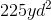.

II) The perimeter of square R is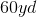.

Statement 2 is sufficient to solve the question, but statement 1 is not sufficient to solve the question.

Both statements taken together are sufficient to solve the question.

Neither statement is sufficient to solve the question. More information is needed.

Statement 1 is sufficient to solve the question, but statement 2 is not sufficient to solve the question.

Each statement alone is enough to solve the question.

Each statement alone is enough to solve the question.

Explanation:

Consider the following equations: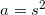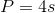Where a is area, p is perimeter, and s is side length

We can find the side length with either our area or our perimeter.

Thus, we only need one statment or the other.

### Example Question #3 : Dsq: Calculating The Length Of The Side Of A Square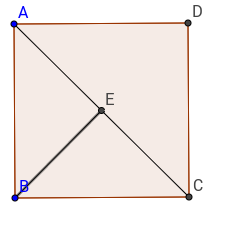What is the length of the side of square, knowing that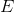is the midpoint of diagonal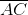?

(1)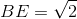(2)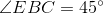Both statements together are sufficient

Statement 2 alone is sufficient

Statements 1 and 2 together are not sufficient

Statement 1 alone is sufficient

Each statement alone is sufficient

Statement 1 alone is sufficient

Explanation:

Since ABCD is a square, we just need to know the length of the diagonale to find the length of the side. BE is half the diagonal, therefore knowing its length would help us find the length of the sides.

Statement 1 tells us the length of BE, therefore, with the formula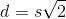whereis the diagonal andthe length of side, we can find the length of the side.

Statement 2 tells us that triangle AEB is isoceles, but it is something we could already have known from the beginning since we are told that E is the midpoint of the diagonal.

Therefore, statement 1 alone is sufficient.

### Example Question #4 : Dsq: Calculating The Length Of The Side Of A Square

Find the area of square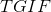.

I)has a diagonal of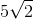inches.

II)has a perimeter ofinches.

Either statement alone is sufficient to answer the question.

Statement I is sufficient to answer the question, but Statement II is not sufficient to answer the question.

Statement II is sufficient to answer the question, but Statement I is not sufficient to answer the question.

Both statements together are needed to answer the question.

Either statement alone is sufficient to answer the question.

Explanation:

To find the area of a square we need to find its side length.

In a square, the diagonal allows us to find the other two sides. The diagonal of a square creates two 45/45/90 triangles with special side length ratios.

I) Gives us the diagonal, which we can use to find the side length, which will then help us find the area.

II) Perimeter of a square allows us to find side length, which in turn lets us find area.

So, either statement is sufficient.

### Example Question #5 : Dsq: Calculating The Length Of The Side Of A Square

Calculate the length of the square.

Statement 1): The area is.

Statement 2): The diagonal is.

Statement 1) ALONE is sufficient, but Statement 2) ALONE is not sufficient to answer the question.

BOTH statements TOGETHER are NOT sufficient, and additional data is needed to answer the question.

EACH statement ALONE is sufficient.

Statement 2) ALONE is sufficient, but Statement 1) ALONE is not sufficient to answer the question.

BOTH statements taken TOGETHER are sufficient to answer the question, but neither statement ALONE is sufficient.

EACH statement ALONE is sufficient.

Explanation:

Statement 1) gives the area of the square.  For all positive real numbers, the formula,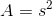, or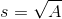, can be used to find either area or side length interchangeably.

Statement 2) mentions that the diagonal is 1, which is a positive real number.  The formula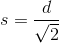, can be used to also find the side length.

Either statement alone is sufficient to solve for the length of the square.

### Example Question #6 : Dsq: Calculating The Length Of The Side Of A Square

Find the length of the quadrilateral.

Statement 1.)  The area of a quadrilateral is.

Statement 2.)  All interior angles of a quadrilateral are right angles.

Statement 1) ALONE is sufficient, but Statement 2) ALONE is not sufficient to answer the question.

BOTH statements taken TOGETHER are sufficient to answer the question, but neither statement ALONE is sufficient.

Statement 2) ALONE is sufficient, but Statement 1) ALONE is not sufficient to answer the question.

BOTH statements TOGETHER are NOT sufficient, and additional data is needed to answer the question.

EACH statement ALONE is sufficient.

BOTH statements TOGETHER are NOT sufficient, and additional data is needed to answer the question.

Explanation:

Statement 1) mentions that the area of a quadrilateral is 4.  This statement is insufficient to solve for the length of the square because the family of quadrilaterals include any 4-sided shape with 4 interior angles.  Examples of quadrilaterals are squares, rectangles, rhombus, and trapezoids, but the quadrilateral is not necessarily a square.

Statement 2) mentions that all four interior angles of a quadrilateral are right angles.  This narrows down the shape to either a square or a rectangle.  Both shapes have 4 right angles, but there is not enough information to determine if the shape is a square or a rectangle.

Therefore, neither statement is sufficient to solve for the length of a quadrilateral.

Tired of practice problems?

Try live online GMAT prep today.